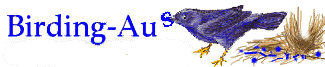birding-aus [Top] [All Lists]

## Is this usual ?

 To: "Peter Ewin" <>, "Birding- aus" <> Is this usual ? Mon, 15 Jun 2009 07:46:07 +0000
 ```Hi all Not sure where the pelicans are but not on Lake Eyre. I'm just on my way home from South Australia on a trip with Outback track Tours to visit Lake Eyre. We did a flight over Lake Eyre last Monday, from Marree in the south to the northern end at the Warburton groove, where Warburton Creek enters the lake. There were about 50 pelicans sitting possibly on nests on one of the two islands, no others across the whole lake. Jill Sent from my BlackBerry® from Optus -----Original Message----- From: Peter Ewin Date: Wed, 10 Jun 2009 12:12:44 To: ; ; ? birding-aus Subject: RE: [Birding-Aus] Is this usual ? Yes what I have been told by guides on the local paddle steamers in Mildura= is that the levels of oxygen in the river fall sufficiently in the cold we= ather that the Bony Bream die=2C and that this attracts birds at this time = of year (particularly Pelicans). Not certain that is the exact answer=2C bu= t something is happening to the fish (maybe just water temperature is too c= old?) and the bird numbers do seem to increase. I was just thinking yesterday as I drove to work over the Mildura Bridge th= at I hadn't noticed a build up of pelicans this year=2C but maybe they are = still up north having too much fun in the Lake Eyre floods to bother about = a few fish in the Murray River. Cheers=2C Peter > From: m("bigpond.com","spanda8"); > To: m("esc.net.au","pratincole");=3B m("vicnet.net.au","birding-aus"); > Subject: RE: [Birding-Aus] Is this usual ? > Date: Wed=2C 10 Jun 2009 12:08:53 +1000 >=20 > Hi Tony >=20 > When we were living in Mildura=2C it was quite common during the cold sea= son > to see significant numbers of Darters=2C along with many=2C many Cormoran= ts=2C > Pelicans and Gulls on the river feeding on what I was told were "Bony Bre= am" > that were "dying?" (perhaps just weakened) in the river. Some cold morni= ngs > it was quite spectacular to see perhaps 200 or more birds fishing just ab= ove > and below the weir. Maybe what you saw also is due to the cold! >=20 > Bob Cook > -----Original Message----- > From: m("vicnet.net.au","birding-aus-bounces"); > m("vicnet.net.au]","[birding-aus-bounces"); On Behalf Of Tony Russell > Sent: Wednesday=2C 10 June 2009 11:01 AM > To: Birds > Subject: [Birding-Aus] Is this usual ? >=20 > Whilst spending a few days in the Renmark - Paringa area last week we > were surprised to find relatively large numbers of Darters. The largest > group of some 20 or so were just below Lock 5 at Paringa=2C but we also > saw individuals and groups of two or three just about everywhere we went > along the river and lagoons. One can usually find a few Darters=2C most > frequently as lone birds=2C along the river but these numbers seemed > exceptional. Any thoughts ? > =20 > Tony. > =3D=3D=3D=3D=3D=3D=3D=3D=3D=3D=3D=3D=3D=3D=3D=3D=3D=3D=3D=3D=3D=3D=3D=3D= =3D=3D=3D=3D=3D=3D=3D > www.birding-aus.org > birding-aus.blogspot.com >=20 > To unsubscribe from this mailing list=2C=20 > send the message: > unsubscribe=20 > (in the body of the message=2C with no Subject line) > to: m("vicnet.net.au","birding-aus-request"); > =3D=3D=3D=3D=3D=3D=3D=3D=3D=3D=3D=3D=3D=3D=3D=3D=3D=3D=3D=3D=3D=3D=3D=3D= =3D=3D=3D=3D=3D=3D=3D >=20 > =3D=3D=3D=3D=3D=3D=3D=3D=3D=3D=3D=3D=3D=3D=3D=3D=3D=3D=3D=3D=3D=3D=3D=3D= =3D=3D=3D=3D=3D=3D=3D > www.birding-aus.org > birding-aus.blogspot.com >=20 > To unsubscribe from this mailing list=2C=20 > send the message: > unsubscribe=20 > (in the body of the message=2C with no Subject line) > to: m("vicnet.net.au","birding-aus-request"); > =3D=3D=3D=3D=3D=3D=3D=3D=3D=3D=3D=3D=3D=3D=3D=3D=3D=3D=3D=3D=3D=3D=3D=3D= =3D=3D=3D=3D=3D=3D=3D _________________________________________________________________ Looking for a new car this winter? Let us help with car news=2C reviews and= more http://a.ninemsn.com.au/b.aspx?URL=3Dhttp%3A%2F%2Fsecure%2Dau%2Eimrworldwid= e%2Ecom%2Fcgi%2Dbin%2Fa%2Fci%5F450304%2Fet%5F2%2Fcg%5F801459%2Fpi%5F1004813= %2Fai%5F859641&_t=3D762955845&_r=3Dtig_OCT07&_m=3DEXT= =============================== www.birding-aus.org birding-aus.blogspot.com To unsubscribe from this mailing list, send the message: unsubscribe (in the body of the message, with no Subject line) to: m("vicnet.net.au","birding-aus-request"); =============================== ``````=============================== www.birding-aus.org birding-aus.blogspot.com To unsubscribe from this mailing list, send the message: unsubscribe (in the body of the message, with no Subject line) to: m("vicnet.net.au","birding-aus-request"); ===============================```
 Current Thread Is this usual ?, Tony Russell Is this usual ?, Bob Cook Is this usual ?, Peter Ewin Is this usual ?, Graham Turner Is this usual ?, jillmolan <= Is this usual ?, Peter Pfeiffer